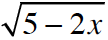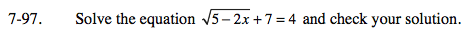Home > INT3 > Chapter 7 > Lesson 7.2.4 > Problem7-97

7-97.

Solve the equation+ 7 = 4 and check your solution. Homework Help ✎Subtract 7 from each side. Do you notice that the radical is equal to a negative value?

What does this mean?